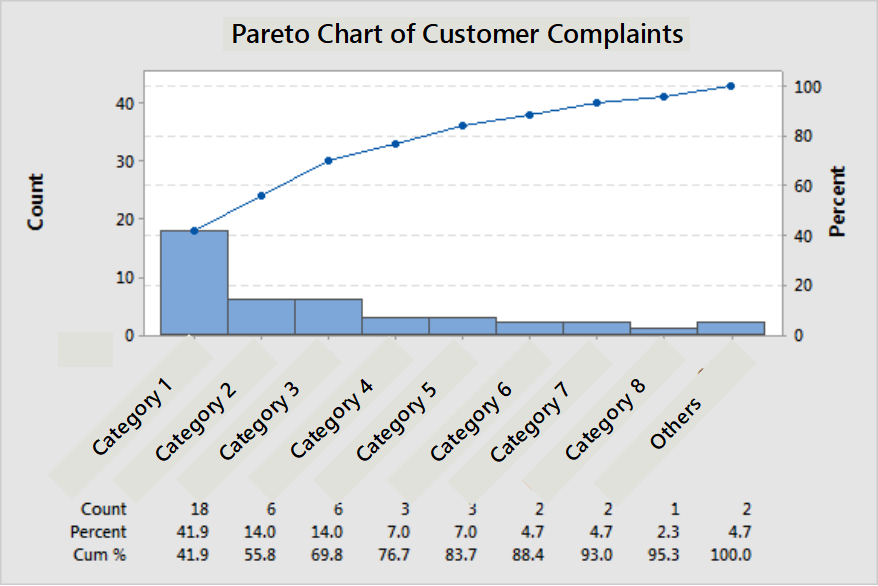### Data Analysis – Minitab TrainingMinitab Training will help you to understand statistics, data analysis and will give you hands-on with Minitab 17. Statistics covered here forms the basis for Data Analytics.

## What is Minitab?

Minitab is a software package that helps us in the analysis of data. It is also extensively used by Six Sigma Experts a is an important part of studying data, particularly in the Measure and Analyse phases of DMAIC. Minitab provides a quick, effective solution for the level of analysis. Let’s understand how to effectively conduct Statistical Data Analysis using Minitab.

## Statistics and DMAIC

This Minitab Training is designed as per DMAIC structure of Six Sigma. Because Six Sigma is an investigative problem-solving methodology and Statistical Data Analysis is the backbone of Six Sigma.

In DMAIC methodology, the problem is defined in the first phase.

### Measure Phase

In Measure Phase, the defined problems are measured in terms of statistics. In this phase, the real world problem gets converted into a statistical problem which is easier to solve. We need to be doubly sure that our data is reliable. Hence, we use Measurement System Analysis to check the reliability of our data sources. And also we arrive at a sampling size and sampling methodology, do collect data,  we screen the data for errors – trends and patterns, typo-errors, the stability of process to validate that collected data represent the process.

We need to fit our data into a statistical model. Mostly we use Normal-Curve Model. So, we check the Normality of our data to understand how much closer it is to the normal curve model.

If our data fits satisfactorily with Normal curve model, then we use this model to calculate Process Capability. We here discuss three methods of calculating process capability, viz., Defects Per Million Opportunities (DPMO), Process Capability Index – Corrected Process Capability Index (Cp & Cpk) and Standard Normal Approximation method. We learn all these tests in the Minitab Training.

### Analyse Phase

With the mathematical problem – usually poor process capability – low capability number, we proceed to Root cause analysis in the Analyse Phase. The root causes of the problem are identified and proved in Analyse Phase using Statistical Tools like Hypothesis Testing, Regression, BoxPlot, Histogram, Pareto, Design of Experiments. In Analyse phase we arrive at statistical solutions to statistical problems. We look at all data analysis tools, namely, Pareto chart, Box-plot, Histogram, Hypothesis testing using the 1-sample t-test, 2-sample t-test, paired t-test, ANOVA, Regression Analysis in this Minitab Training.

### Control Phase

We convert the statistical solutions into real life solutions at Improve Phase. Once the improvements are achieved and the problem is solved, we move on to make the changes getting into practice through Control Phase. As the saying goes, focus on your duty and results will follow, we will control the factors using control charts to keep the problem away. We understand uses of various control charts and generation of charts using Minitab in this Minitab Training.## Training Contents

### Basics of Statistics

• #### Data Collection & Display

• Frequency Table
• Concentration Diagrams
• Stem and Leaf Diagrams
• Frequency Distribution
• Histogram
• #### Descriptive Statistics

• Definitions
• Population and Sample
• Why do we need samples?
• Different methods of sampling
• Estimating Sample Size
• #### Properties of data

• Central Tendency
• Mean, Median, Mode & Quartiles
• Dispersion
• Range, Variance, Standard Deviation & IQR
• #### Introduction to Minitab

• Descriptive Statistics using Minitab
• #### Inference from Data

• Inferential statistics
• Probability Distributions
• Normal Distribution
• #### Measurement System Analysis (based on AIAG Guidelines)

• Why MSA?
• Measurement Errors
• Accuracy and Precision of Measurements
• Measurement Linearity
• Gage R&R
• Attribute Agreement Analysis
• #### Understanding Behaviour of collected data

• Trends & Patterns in Data
• Trend Analysis
• Variation & its consequences
• Stability Analysis
• Test for Normality
• #### Process Capability

• Cp & Cpk
• DPMO
• Standard Normal Distribution (Z)
• #### Basic Data Analysis Tools

• Histogram
• Pareto Analysis
• Box Plot

### Minitab Tools

• Descriptive Statistics
• Gage R & R
• AAA
• Time Series Chart
• Control Charts
• Normality Test
• Capability Analysis
• Histogram
• Pareto Chart
• Box Plot

### Analyse

• t – Test
• F – Test
• ANOVA
• Chi Square
• #### Regression Analysis

• Regression and Correlation
• Determination Coefficient
• Simple Linear Regression
• Curvilinear Regression
• Multiple Linear Regression

### Control Phase

• Control-Charts
• Individual Values
• Variables
• Attributes

### Minitab Tools

• 1 Sample t Test
• 2 Sample t Test
• Paired t Test
• ANOVA
• Chi Square
• Regression
• ANOVA – GLM
• DOE
• Factorial Design
• Factorial Analysis
• Response Optimiser
• I Chart
• I – MR Chart
• X bar R Chart
• P Chart
• NP Chart
• U Chart
• C Chart